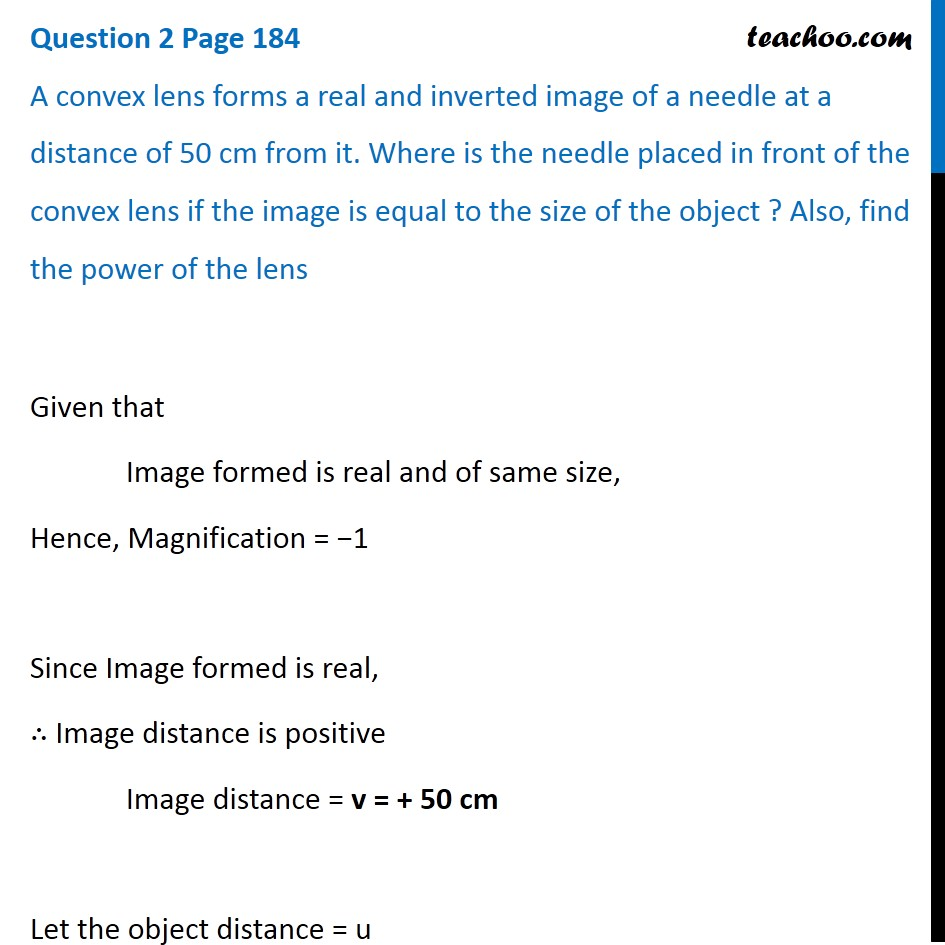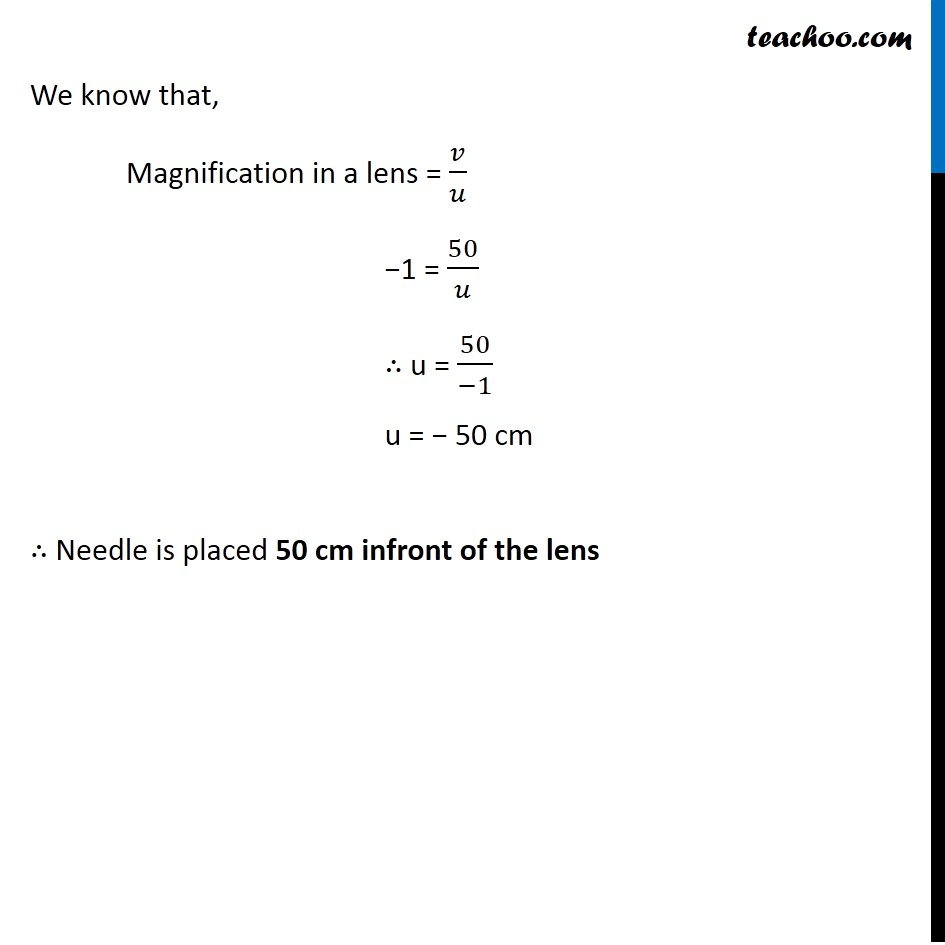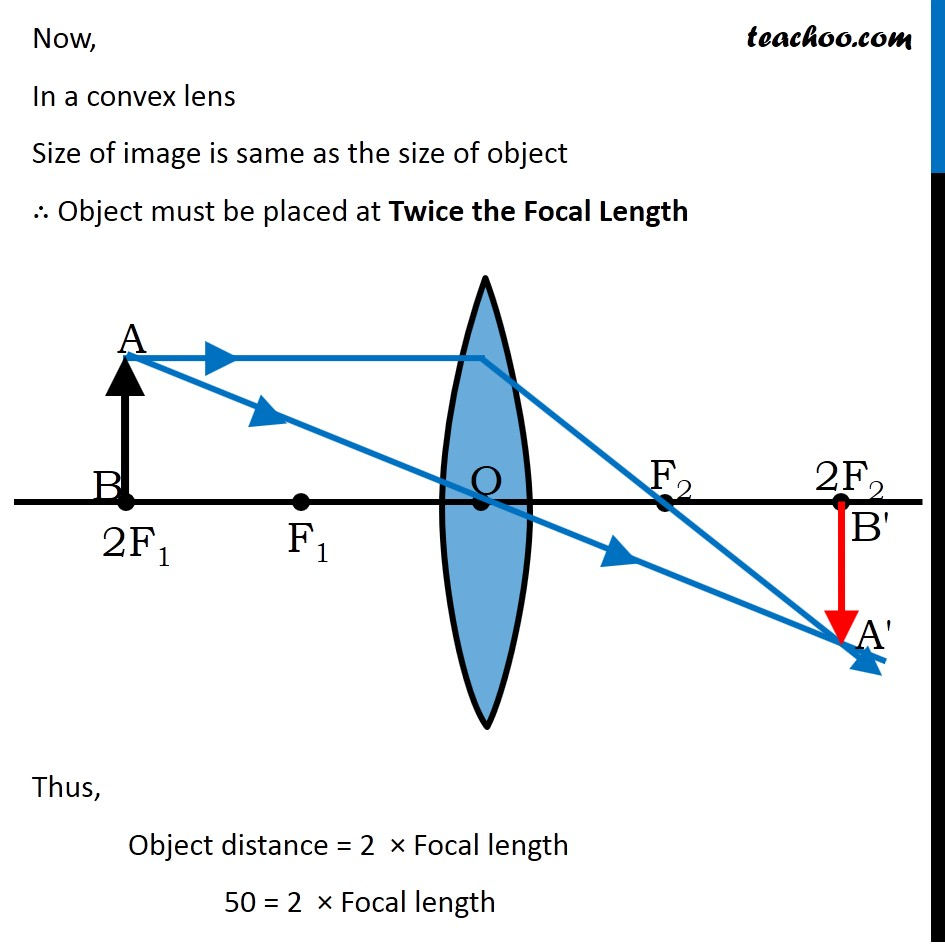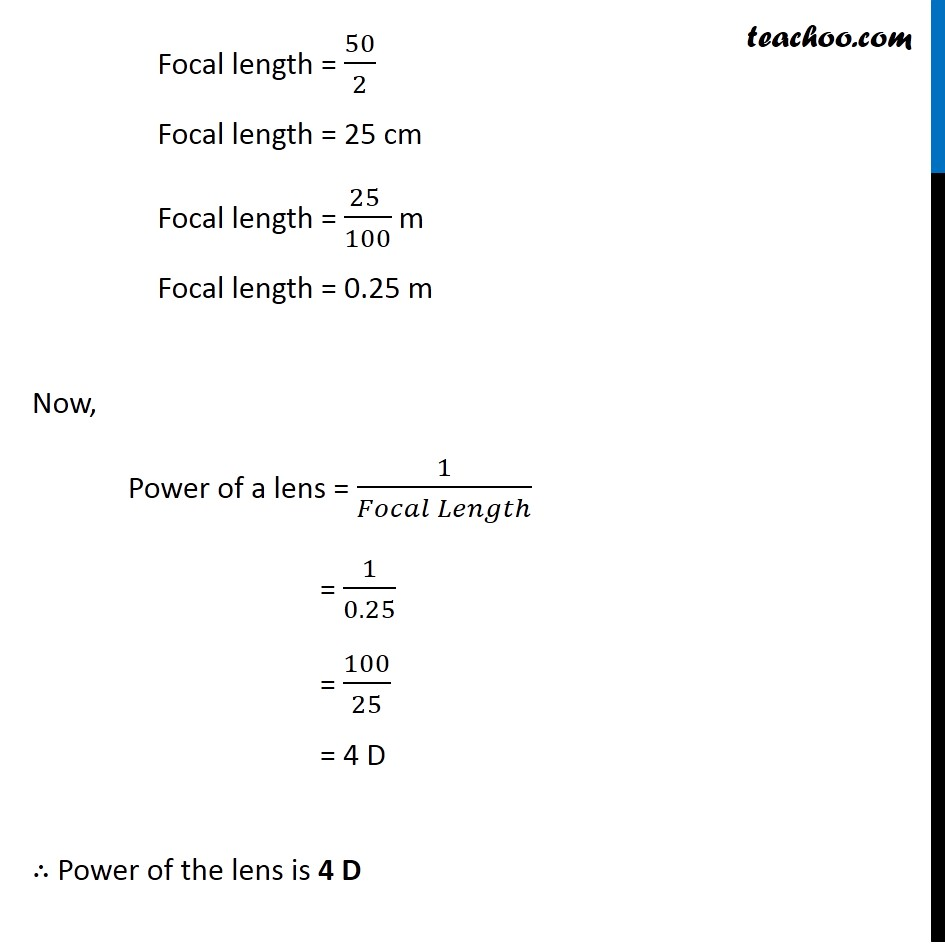Questions from Inside the chapter

Class 10
Chapter 10 Class 10 - Light - Reflection and Refraction

## A convex lens forms a real and inverted image of a needle at a distance of 50 cm from it. Where is the needle placed in front of the convex lens if the image is equal to the size of the object? Also, find the power of the lensLearn in your speed, with individual attention - Teachoo Maths 1-on-1 Class

### Transcript

Question 2 Page 184 A convex lens forms a real and inverted image of a needle at a distance of 50 cm from it. Where is the needle placed in front of the convex lens if the image is equal to the size of the object ? Also, find the power of the lens Given that Image formed is real and of same size, Hence, Magnification = −1 Since Image formed is real, ∴ Image distance is positive Image distance = v = + 50 cm Let the object distance = u We know that, Magnification in a lens = 𝑣/𝑢 −1 = 50/𝑢 ∴ u = 50/(−1) u = − 50 cm ∴ Needle is placed 50 cm infront of the lens Now, In a convex lens Size of image is same as the size of object ∴ Object must be placed at Twice the Focal Length Thus, Object distance = 2 × Focal length 50 = 2 × Focal length Now, In a convex lens Size of image is same as the size of object ∴ Object must be placed at Twice the Focal Length Thus, Object distance = 2 × Focal length 50 = 2 × Focal length Focal length = 50/2 Focal length = 25 cm Focal length = (25 )/100 m Focal length = 0.25 m Now, Power of a lens = 1/(𝐹𝑜𝑐𝑎𝑙 𝐿𝑒𝑛𝑔𝑡ℎ) = 1/0.25 = 100/25 = 4 D ∴ Power of the lens is 4 D